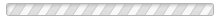# Linux环境C语言编程基础(91arm.com)

1、一个程序的执行是从( ).*
2、( )是C语言合法的常量。*
3、以下叙述正确的是( ) *
4、以下叙述不正确的是 ( ) *
5、int x=3，y=2；则表达式x+=x*=y+8的值为( )*
6、设x=2.7，a=8，y=4.9，算术表达式x+a%3*(int)(x+y)%5/3的值为( )*
7、执行下面两个语句后  char c1=98;  printf（“%d %c”，c1，c1-32）； 输出的结果为( )*
8、执行下面语句y=10；x=y++；后的结果为( ) *
9、char w；int x；float y；double z；则表达式w*x+z-y值的数据类型是( )*
10、C语言中要求操作数必须是整数的运算符是( )*
11、用scanf(“%d，%d”，&a，&b)输入数据时，下面输入法中正确的是( )*
12、以下关于运算符优先顺序的描述中正确的是( )*
13、下列程序段中，功能与其它程序段不同的( )*
14、C语言规定else总是与 ( ) 配对。*
15、设x和y均为int型变量，则语句x+=y；y=x－y；x－=y；的功能是( )*
16、变量的指针，其含义是指该变量的( )*
17、以下函数调用语句中含有( )个实参。*
18、以下正确的描述是( )*
19、int a={2,3,4},I;  i=a*a+a*a;  i的值为( ) 。*
20、以下正确的函数定义形式是 ( )*
21、C语言允许函数值类型缺省定义，此时该函数值隐含的类型是( )*
22、若有定义int a={1,2,3};请问a[a[a]]的值是( )。*
23、以下正确的描述是( )*
24、以下不正确的说法是( )*
25、已有定义：int x=3,y=4,z=5; 则表达式!(x+y)+z-1&&y+z/2的值是( ) 。*
26、判断字符串是否相等，应当使用( )*
27、以下程序运行结果是( )。
main()
{char s=”1234567”,*p=s;
int i;
i=*(p+5)-*(p+2);
printf(“%d\n”,i*strlen(s));
}
*
28、以下程序运行结果是( ) 。
main()
{
int a[]={1,2,3,4,5,6,7,8,9},i,j,k;
for(i=0,j=8;i
{k=a[i];a[i]=a[j];a[j]=k;}
printf(“%d\n”,a+a);
}

*
29、以下程序运行结果是( ) 。
main()
{
int x[]={1,2,3},s,*p=x,i;
for(i=0,s=1;i<3;i++,p++)
s*=*p;
printf(“%d\n”,s);
}
*
30、以下程序运行结果是( )。
main()
{
char s=”I am a student”,c;
int i,num=0,word=0;
for(i=0;c=s[i];i++)
if(c==’’) word=0;
else if(word==0)
{word=1;num++;}
printf(“%d\n”,num);
}*问卷星 提供技术支持